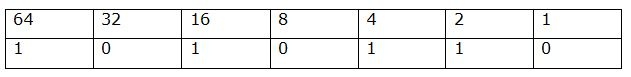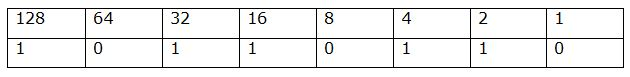# IBPS RRB PO Mains Reasoning Ability Questions 2019 (Day-05)

Dear Aspirants, Our IBPS Guide team is providing new series of Reasoning Questions for IBPS RRB PO Mains 2019 so the aspirants can practice it on a daily basis. These questions are framed by our skilled experts after understanding your needs thoroughly. Aspirants can practice these new series questions daily to familiarize with the exact exam pattern and make your preparation effective.

Check here for IBPS RRB PO Mains Mock Test 2019

Check here for IBPS RRB Clerk Mains Mock Test 2019

Check here for IBPS PO Prelims Mock Test 2019

### Click Here to Subscribe Crack High Level Puzzles & Seating Arrangement Questions PDF 2019 Plan

[WpProQuiz 6860]

Directions (1-4): Study the following information carefully and answer the questions given below.

When a number arrangement machine is given an input of a line of numbers, it arranges them by following a particular rule. The following is an illustration of an input and its rearrangement.

Input:   67    52    48    13     61      82      23

Step I: 201   26    24    39    123     41      69

Step II:     175   2     15    84      82     28

Step III:     35    2      5     32     16     16

Step IV:      8     2      5      5       7       7

Step V:        4    1     2.5    2.5    3.5   3.5

Step V, is the last step of the above arrangement as the intended arrangement is obtained.

As per the rules followed in the given steps find out the appropriate steps for the given input:

Input:  79   48    31    16    22    81    38

1) What is the sum of third element from the left end in step II and fourth element from the right end in step IV?

a) 100

b) 89

c) 87

d) 81

e) None of these

2) What is the product of second element from the right end in step III and fifth element from the left end in step IV?

a) 48

b) 96

c) 36

d) 40

e) None of these

3) What is the difference between second element from the right end in step I and fourth element from the left end in step V?

a) 229.5

b) 240.5

c) 236.5

d) 241.5

e) None of these

4) What is the sum of all the elements in step V?

a) 15

b) 16

c) 13

d) 14

e) None of these

Directions (5-8): Each of the questions below consists of a question and two statements numbered I and II given below it. You have to decide whether the data given in the statements are sufficient to answer the question. Read both statements and choose the most appropriate option

a) Only Statement I is sufficient to answer

b) Only Statement II is sufficient to answer

c) Either Statement I or Statement II is sufficient to answer.

d) Neither Statement I nor Statement II is sufficient to answer.

e) Both Statement I and Statement II are sufficient to answer.

5) Seven persons G, M, H, S, D, F and R attend the lecture in a week starting from Monday and ending on Sunday. How many persons attend the lecture between G and D?

I) R attends the lecture after M. More than three persons attend the lecture between M and R. Three persons attend the lecture between H and D. S attends the lecture immediately before F.

II) Two persons attend the lecture between F and H. M attends the lecture on Monday. Only two persons attend the lecture after F. S attends the lecture immediately after G and does not attend the lecture on last day. R attends the seminar after D.

6) Eight persons P, Q, R, S, T, U, V and W are sitting around a circular table facing away from the centre. Who among the following person sits third to the right of S?

I) Two persons sit between P and T. R is neither an immediate neighbour of P nor an immediate neighbour of T.Q sits second to the right of R.

II) U sits opposite to R. As many persons sit between S and W sits between S and V. Q and S are not immediate neighbours.

7) Eight persons A, B, C, D, E,F, G and H are sitting in a row facing north. Person’s name starts with consecutive alphabet does not sit next to each other. How many persons sit between G and E?

I) Two persons sit between A and C. C sits second from one of the ends. Only one person sits between B and G. D and B are not immediate neighbours. F sits to the right of C. H does not sit at any of the extreme ends.

II) More than three persons sit between C and E. B sits to the right of D. H sits left of A.

8) Seven persons H, L, K, B, S, W and D were born in different months March, May, June, August, September, October and November but not necessarily in the same order. Who was born in June?

I) Three persons were born between B and H. H was born after B. S was born immediately before D but not immediately after B.

II) As many persons born before K were born after H. W was born after L.

Directions (9-10): Study the following information carefully and answer the questions given below.

In a certain code, the symbol for 0 is £ and for 1 is &. There are no other symbols for all other numbers greater than one. The numbers greater than 1 are to be written only by using the two symbols given above. The value of the symbol for 1 doubles itself every time it shifts one place to the left. Study the following examples:

0 is written as £

1 is written as &

2 is written as &£

3 is written as &&

4 is written as &££ and so on.

9) What is the code for ‘86’?

a) &£&£&££

b) &£&££&£

c) &£&£&&£

d) £&&£&&£

e) None of these

10) Which of the following number will be represented as& £&& £&&£?

a) 192

b) 182

c) 124

d) 167

e) None of these

Directions (1-4):

Step I: If it is an odd number, then it is should be multiplied by 3, else it should be divided by 2

Step II: Difference between 1st and 2nd, 2nd and 3rd, 3rd and 4th

Step III: Multiply all the digits in a number

Step IV: Sum of the digits in a number

Step V: Each number is divided by 2

Input:  79   48    31    16    22    81    38

Step I: 237   24    93     8      11    243   19

Step II:     213   69    85    3     232    224

Step III:     6      54    40    3     12      16

Step IV:      6      9      4      3     3        7

Step V:       3      4.5    2    1.5   1.5   3.5

3rd element from the left end of step II= 85

4th element from the right end of step IV= 4

Sum = 85+4= 89

Option b is the correct answer.

2nd element from the right end in step III= 12

5th element from the left end in step IV= 3

Product= 12*3= 36

Option c is the correct answer.

2nd element from the right end in step I= 243

4th element from the left end in step V= 1.5

Difference= 243-1.5= 241.5

Option d is the correct answer.

Sum of all elements in step V=3+4.5+2+1.5+1.5+3.5= 16

Option b is the correct answer.

Only II is sufficient.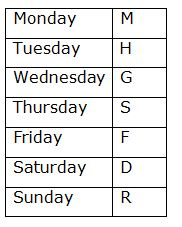Therefore, b is the correct answer.

From I and II,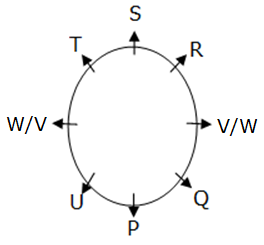Therefore, e is the correct answer.

From I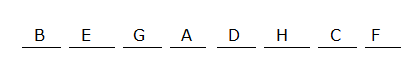Therefore, a is the correct answer.

From I and II,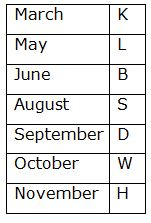Therefore, e is the correct answer.

Directions (9-10):